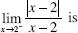# AP Calculus AB Practice Test 27

### Test Information9 questions18 minutes

Calculator Disallowed

1. Use the following table, which shows the values of differentiable functions f and g.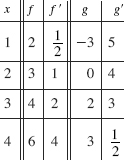If H(x) = f -1(x), then H (3) equals

2. The total area of the region bounded by the graph of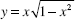and the x-axis is

3. The graph of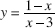is concave upward when

4. As an ice block melts, the rate at which its mass, M, decreases is directly proportional to the square root of the mass. Which equationdescribes this relationship?

5. The average (mean) value of tan x on the interval from x = 0 to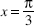is

6.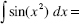7. Water is poured at a constant rate into the conical reservoir shown in the figure.If the depth of the water, h, is graphed as a function of time, the graph is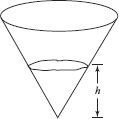8. If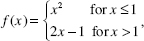then

9.